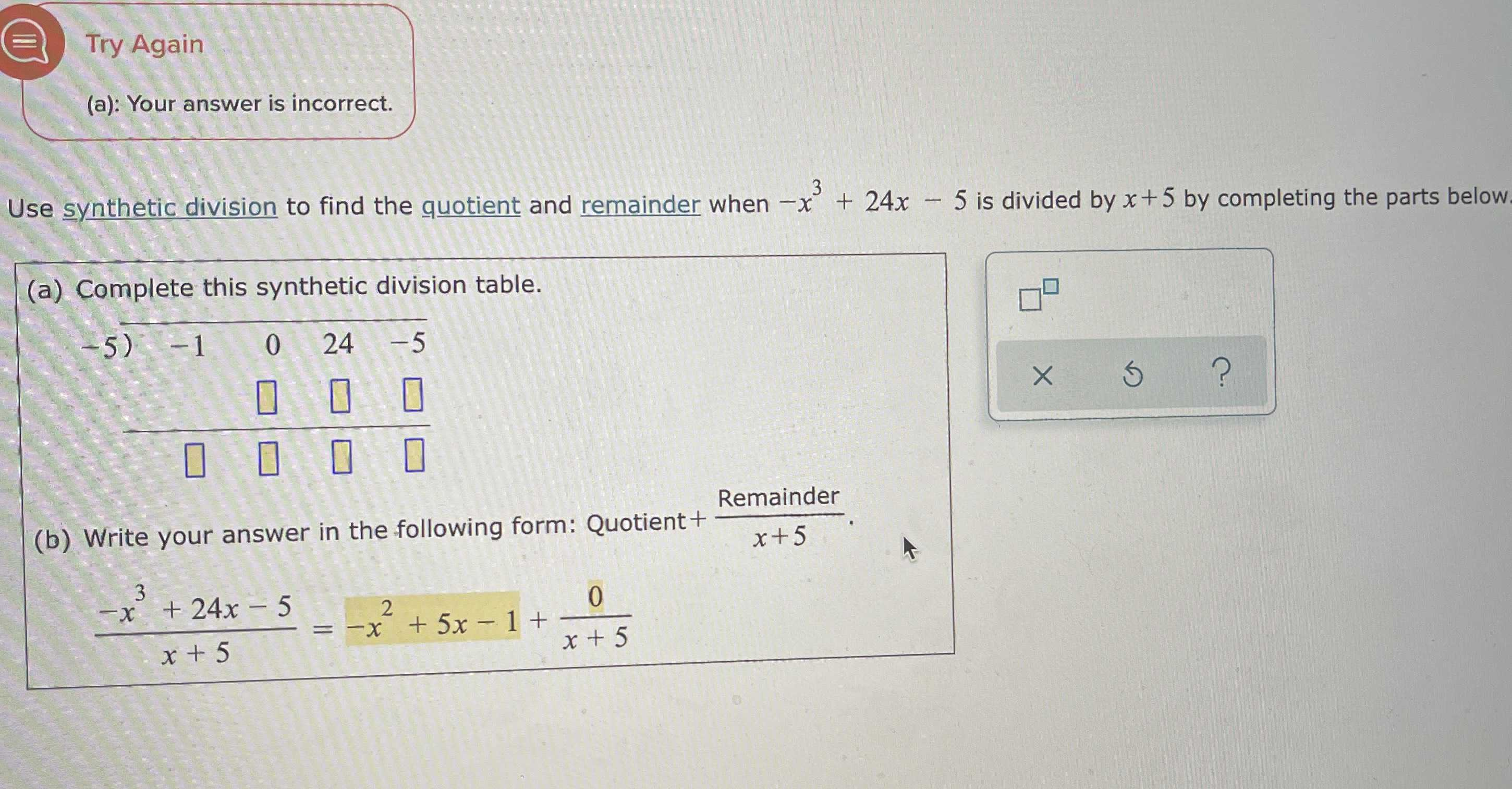### ¿Todavía tienes preguntas de matemáticas?

Pregunte a nuestros tutores expertos
Algebra
PreguntaUse synthetic division to find the quotient and remainder when $$- x ^ { 3 } + 24 x - 5$$ is divided by $$x + 5$$ by completing the parts below.

(a) Complete this synthetic division table.

(b) Write your answer in the following form: Quotient $$+$$ $$\frac{Remainder}{x+ 5}$$